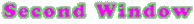Print out this form and use it to record your values. See if you can get an idea of the range of wavelength values for each type of light. Can you determine the boundary numbers which are typically used to separate each wavelength type from the ones next to it?

NAME(S):
WAVELENGTH VALUES IN ANGSTROMS

visible light

longest wavelength value =
value 2=
value 3=
shortest wavelength value =

gamma rays

longest wavelength value=
value 2=
value 3=
shortest wavelength value=

x-rays

longest wavelength value=
value 2=
value 3=
shortest wavelength value=

longest wavelength value=
value 2=
value 3=
shortest wavelength value=

extreme ultraviolet

longest wavelength value=
value 2=
value 3=
shortest wavelength value=

infrared

longest wavelength value=
value 2=
value 3=
shortest wavelength value=

ultraviolet

longest wavelength value=
value 2=
value 3=
shortest wavelength value=

Now, can you order the wave types correctly on the electromagnetic spectrum from shortest wavelength light type to longest? Try it! Fill in the Electromagnetic Spectrum Chart with your results.

Copyright © 1996-2001, The Regents of the University of California. For permission, email outreach@ssl.berkeley.edu.